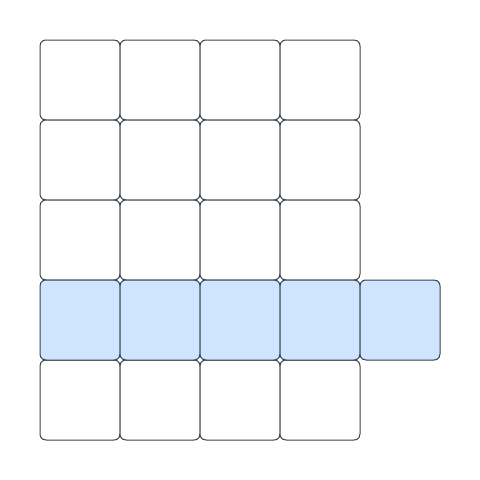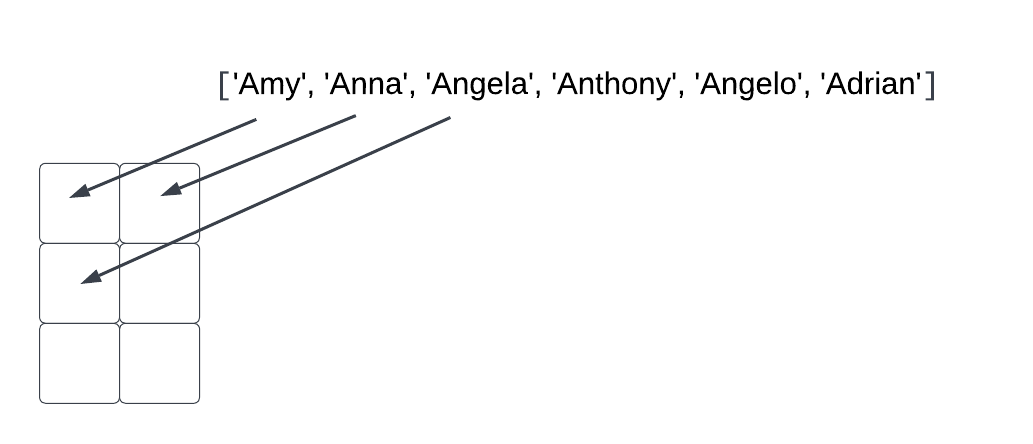Computer Science

# Grids

A grid is a list of lists. You can think of this as a two-dimensional structure. For example, if we have this grid:

``````numbers = [
[1, 2, 3],
[4, 5, 6]
]``````

Then we can visualize it like this:In some programming languages you will see these called an array. In math you might call this a matrix.

## Printing a grid

It helps to think of a grid as a list of rows. So we can print out a grid like this:

``````def print_grid(grid):
for row in grid:
for item in row:
print(item, end=' ')
print()``````

If we call it like this:

``````numbers = [
[1, 2, 3],
[4, 5, 6]
]

print_grid(numbers)``````

then we get:

``````1 2 3
4 5 6 ``````

Go back and look at this example code. We are using `print(item, end=' ')` to print each item in each row. This will print one of the numbers, followed by a space instead of a newline. This ensures all of the numbers on the same row appear on the same line: `1 2 3`. After we done with all of the numbers in a row, we call `print()`. We don’t tell it to print anything, but print always ends with a newline, unless override this with `end`, so `print()` by itself prints a newline to end the row.

So technically, the code above prints:

``````1<space>2<space>3<space><newline>
4<space>5<space>6<space><newline>``````

## Copying a grid

Let’s write a function that copies a grid:

``````def copy_grid(grid):
new_grid = []
for row in grid:
new_row = []
for item in row:
new_row.append(item)
new_grid.append(new_row)
return new_grid``````

We need to be sure to create a new grid, using the `new_grid` variable and initializing it to an empty list `[]`. Then, each time we create a new row that is also initialized with `[]`. We append each item in a row to a new row, then append each row to the new grid.

Step through every line of this in the debugger to be sure you understand what it is doing.

If we call this function with:

``````numbers = [
[1, 2, 3],
[4, 5, 6]
]
more_numbers = copy_grid(numbers)
print(more_numbers)``````

We will see:

``[[1, 2, 3], [4, 5, 6]]``

Remember, a grid is just a nested set of lists. Each nested list is a row in the grid.

## Creating an empty grid

Sometimes you want to create an empty grid, meaning a grid filled with `None` in every row and column. Here is a function that will do that:

``````def empty_grid(num_rows, num_columns):
new_grid = []
for row in range(num_rows):
new_row = []
for column in range(num_columns):
new_row.append(None)
new_grid.append(new_row)
return new_grid``````

The function takes two parameters — `num_rows` and `num_columns`. This tells us how many rows and columns we need to create in the grid.

Like when we copy a grid, above, we create an empty grid, initialized to `[]`. Each time we create a new row, we likewise initialize it to `[]`. We append `None` to the row, one for each column. We append each row to the new grid.

We can call this function:

``print(empty_grid(3, 2))``

And this will print an empty grid of 3 rows and 2 columns:

``[[None, None], [None, None], [None, None]]``

## Testing for a jagged grid

A jagged grid is one where the rows are not all the same length.

Let’s write a function that returns `True` if a grid is jagged. Think about how you might solve this problem. Out of all of the rows of a grid, there might be just one that is too long:Or there might be many rows that are too long! Or maybe there are none!

We can loop through the grid and store the length of the first row into a variable called `size`. Then for each subsequent row, we can check if the length of that row is equal to `size`. If it is not, we can return early with `True`. Otherwise, after we loop over the entire grid, we can return `False`.

Here is some code that implements this algorithm:

``````def is_jagged(grid):
size = None
for row in grid:
if size is None:
size = len(row)
elif len(row) != size:
return True
return False``````

Notice that this kind of algorithm is a lot like finding the maximum of a list of numbers. We start with `size` equal to `None`. This means that the first time we check a row, we will set `size` equal to the length of that row. For every subsequent row, we will compare the length of the new row to `size`.

We can call this function to check for a jagged grid:

``````grid_a = [
[1, 2, 3, 4],
[5, 6, 7]
]
print(is_jagged(grid_a))

grid_b = [
[1, 2],
[3, 4],
[5, 6],
[7, 8]
]
print(is_jagged(grid_b))``````

This should print `True` and then `False`.

## Parsing grids

It will be handy to load a grid from a file. Lets imagine an input file, called `grid.txt` contains this:

``````4 5 6 7
8 9 10 11
1 2 3 4``````

Each row is on a separate line of the file. We have a grid containing only integers. Each integer is separated by spaces. This means we can use `split()` to get each number in each row, and then append the number to the grid. We have to be sure to convert from strings to integers as we do this.

Here is some code that reads a grid from a file:

``````def load_grid(filename):
grid = []
with open(filename) as file:
for line in file:
row = []
for item in line.strip().split():
row.append(int(item))
grid.append(row)
return grid``````

We use `line.strip().split()` to first strip off the newline at the end of the line, and then split the line into a list of strings based on the space separator. We can then append each item to its row with `row.append(int(item))`, where we are sure to convert to integers first.

Let’s load `grid.txt` into a grid and then print it:

``````grid = load_grid('grid.txt')
print(grid)``````

This should print:

``[[4, 5, 6, 7], [8, 9, 10, 11], [1, 2, 3, 4]]``

## Converting a list to a grid

Sometimes you are given just a long list of data:

``['Amy', 'Anna', 'Angela', 'Anthony', 'Angelo', 'Adrian']``

and you need to convert it to a grid. let’s write a function that does this. Whoever calls our function specifies the number of rows and the number of columns. We will assume that the number of items in the list fits the dimensions of our grid perfectly.

We need to plan this out to be sure we get it right. Let’s say we want to convert the above list into a grid that has 3 rows and 2 columns. Then:This means `row 0` has items 0 and 1 from the list, `row 1` has items 2 and 3, and the `row 2` has items 4 and 5. If we are in row `i` and column `j`, then we can calculate the item we need to place here as `i * num_columns + j`. Try out this math and be sure it is right.

If we are in row `1`, column `1`, then we should store item `1 * 2 + 1 = 3` from the list.

Here is code to do this:

``````def to_grid(items, num_rows, num_columns):
grid = []
for i in range(num_rows):
row = []
for j in range(num_columns):
row.append(items[i * num_columns + j])
grid.append(row)
return grid``````

If we run this:

``````names = ['Amy', 'Anna', 'Angela', 'Anthony', 'Angelo', 'Adrian']

name_grid = to_grid(names, 3, 2)
print_grid(name_grid)``````

We get:

``````Amy Anna
Angela Anthony